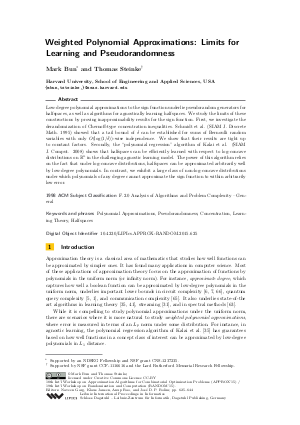Document# Weighted Polynomial Approximations: Limits for Learning and Pseudorandomness

### Authors Mark Bun, Thomas Steinke## File

LIPIcs.APPROX-RANDOM.2015.625.pdf
• Filesize: 0.52 MB
• 20 pages

## Cite As

Mark Bun and Thomas Steinke. Weighted Polynomial Approximations: Limits for Learning and Pseudorandomness. In Approximation, Randomization, and Combinatorial Optimization. Algorithms and Techniques (APPROX/RANDOM 2015). Leibniz International Proceedings in Informatics (LIPIcs), Volume 40, pp. 625-644, Schloss Dagstuhl - Leibniz-Zentrum für Informatik (2015)
https://doi.org/10.4230/LIPIcs.APPROX-RANDOM.2015.625

## Abstract

Low-degree polynomial approximations to the sign function underlie pseudorandom generators for halfspaces, as well as algorithms for agnostically learning halfspaces. We study the limits of these constructions by proving inapproximability results for the sign function. First, we investigate the derandomization of Chernoff-type concentration inequalities. Schmidt et al. (SIAM J. Discrete Math. 1995) showed that a tail bound of delta can be established for sums of Bernoulli random variables with only O(log(1/delta))-wise independence. We show that their results are tight up to constant factors. Secondly, the “polynomial regression” algorithm of Kalai et al. (SIAM J. Comput. 2008) shows that halfspaces can be efficiently learned with respect to log-concave distributions on R^n in the challenging agnostic learning model. The power of this algorithm relies on the fact that under log-concave distributions, halfspaces can be approximated arbitrarily well by low-degree polynomials. In contrast, we exhibit a large class of non-log-concave distributions under which polynomials of any degree cannot approximate the sign function to within arbitrarily low error.
##### Keywords
• Polynomial Approximations
• Pseudorandomness
• Concentration
• Learning Theory
• Halfspaces

## Metrics

• Access Statistics
• Total Accesses (updated on a weekly basis)
0